Ch 1. Stress and Strain Multimedia Engineering Mechanics NormalStress Shear andBearing Stress NormalStrain Hooke'sLaw ThermalEffects IndeterminateStructures
 Chapter 1. Stress/Strain 2. Torsion 3. Beam Shr/Moment 4. Beam Stresses 5. Beam Deflections 6. Beam-Advanced 7. Stress Analysis 8. Strain Analysis 9. Columns Appendix Basic Math Units Basic Equations Sections Material Properties Structural Shapes Beam Equations Search eBooks Dynamics Fluids Math Mechanics Statics Thermodynamics Author(s): Kurt Gramoll ©Kurt GramollMECHANICS - CASE STUDY SOLUTION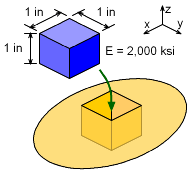1.0 inch Specimen Cube Inserted into Test Frame A simple cube of material is inserted into a snug fitting test frame and then a 3,000 lb load is applied on the top surface. The sides of the test frame are stiff and will not deflect at the test load level. Also, the sides are smooth so there is no friction forces as the specimen is loaded. The objective in testing the specimen is to determine the Poisson's ratio of the material. The Young's modulus, E, is known, 2,000 ksi. Also, the total deflection after loading is 0.001 inches. Determining Poisson's ratio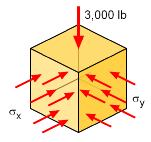Cube Under Loading A good place to start solving this problem is the full 3D Hooke's law equations.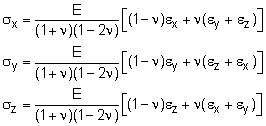While these look complex, many terms are easily determined. The vertical stress can be calculated as      σz = F/A = 3000 lb/(1 in)2 = 3,000 psi = 3.0 ksi The other two horizontal stresses are unknown, but must be the same due to symmetric geometry and loading. Thus,      σx = σy = p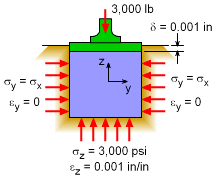Problem Diagram Next, the three strains can be determined. The vertical strain can be found since the deflection is known, giving      εz = δ/L = (0.001 in) / (1.0 in) = 0.001 The other two strains are also known since the outside walls do not deflect (due to their high stiffness). Thus,      εx = εy = 0.0 Also, Young's modulus is known, E = 2,000 ksi.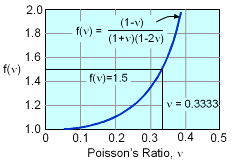Graph of Function f(ν) = (1-ν) / [(1+ν)(1-2ν)] Simplifying the 3D equations to two equations give,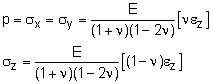Next, substituting specific values reduce these equations to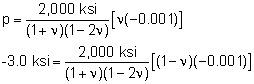The negative signs represent compression for both strain and stress. There are now two unknowns, p and ν. The second equation can be rearranged to give,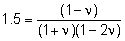This can be manipulated to give      3ν2 + 0.5ν - 0.5 = 0 Using the quadratic equation, the positive root gives      ν = 0.3333 This equation can also be solved numerically by graphical methods or by using numerical programs like MathCad, Mathematica, MathLab, or even Excel. The equation is graphed at the left which also gives      ν = 0.3333 Stresses The stresses in the x and y directions can now be determined,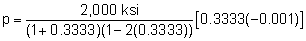p = σx = σy = -1.5 ksi = 1,500 psi

Practice Homework and Test problems now available in the 'Eng Mechanics' mobile app
Includes over 400 problems with complete detailed solutions.
Available now at the Google Play Store and Apple App Store.